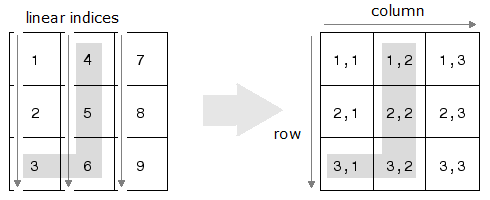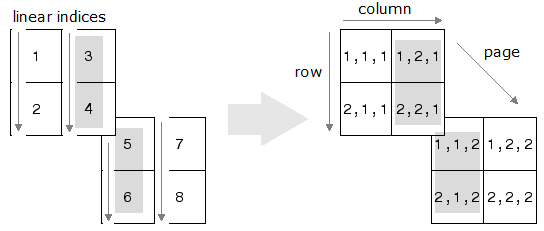# ind2sub

Convert linear indices to subscripts

## Syntax

``[row,col] = ind2sub(sz,ind)``
``[I1,I2,...,In] = ind2sub(sz,ind)``

## Description

example

````[row,col] = ind2sub(sz,ind)` returns the arrays `row` and `col` containing the equivalent row and column subscripts corresponding to the linear indices `ind` for a matrix of size `sz`. Here `sz` is a vector with two elements, where `sz(1)` specifies the number of rows and `sz(2)` specifies the number of columns. ```

example

````[I1,I2,...,In] = ind2sub(sz,ind)` returns `n` arrays `I1,I2,...,In` containing the equivalent multidimensional subscripts corresponding to the linear indices `ind` for a multidimensional array of size `sz`. Here `sz` is a vector with `n` elements that specifies the size of each array dimension.```

## Examples

collapse all

Convert the linear indices `[3 4 5 6]` to row and column subscripts in a 3-by-3 matrix. The mapping from linear indices to subscripts (indexing by position) is illustrated in the following.Create input vectors and perform the conversion.

```ind = [3 4 5 6]; sz = [3 3]; [row,col] = ind2sub(sz,ind)```
```row = 1×4 3 1 2 3 ```
```col = 1×4 1 2 2 2 ```

Convert the linear indices `[3 4 5 6]` to subscripts in a 2-by-2-by-2 array. The mapping from linear indices to subscripts (indexing by position) for a 2-by-2-by-2 array can be illustrated as in the following.Create input vectors and perform the conversion.

```ind = [3 4 5 6]; sz = [2 2 2]; [I1,I2,I3] = ind2sub(sz,ind)```
```I1 = 1×4 1 2 1 2 ```
```I2 = 1×4 2 2 1 1 ```
```I3 = 1×4 1 1 2 2 ```

Convert a linear index of a 3-D array to a subscript index.

Create an array, and find the subscript index corresponding to the 14 th element of the array.

```A = rand(3,4,2); [row,col,page] = ind2sub(size(A),14)```
```row = 2 ```
```col = 1 ```
```page = 2 ```

Check that both index versions refer to the same element of the array.

`A(14)`
```ans = 0.4854 ```
`A(row,col,page)`
```ans = 0.4854 ```

When using `ind2sub` for an N-dimensional array, you would typically supply N output arguments for each dimension of the matrix. This example shows the different results when you return fewer output arguments for a 3-dimensional array.

Create the input arguments needed to convert the linear indices 1 through 8 for a 3-dimensional array with size 2-by-2-by-2.

```ind = 1:8; sz = [2 2 2];```

Specify three output arguments when using `ind2sub` to return the row, column, and page subscripts for the 2-by-2-by-2 array.

`[row,col,page] = ind2sub(sz,ind)`
```row = 1×8 1 2 1 2 1 2 1 2 ```
```col = 1×8 1 1 2 2 1 1 2 2 ```
```page = 1×8 1 1 1 1 2 2 2 2 ```

If you specify only two output arguments, `ind2sub` ignores the third dimension of the array and returns subscripts for a 2-dimensional array with size 2-by-4 instead.

`[row,col] = ind2sub(sz,ind)`
```row = 1×8 1 2 1 2 1 2 1 2 ```
```col = 1×8 1 1 2 2 3 3 4 4 ```

If you specify only one output argument, `ind2sub` ignores the second and third dimensions of the array and returns subscripts for a 1-dimensional array with size 1-by-8 instead.

`row = ind2sub(sz,ind)`
```row = 1×8 1 2 3 4 5 6 7 8 ```

## Input Arguments

collapse all

Size of array, specified as a vector of positive integers. Each element of this vector indicates the size of the corresponding dimension. For example, ```[2 3 4]``` defines a 2-by-3-by-4 array.

Data Types: `single` | `double` | `int8` | `int16` | `int32` | `int64` | `uint8` | `uint16` | `uint32` | `uint64`

Linear indices, specified as a scalar, vector, matrix, or multidimensional array.

Data Types: `single` | `double` | `int8` | `int16` | `int32` | `int64` | `uint8` | `uint16` | `uint32` | `uint64`

## Output Arguments

collapse all

Row subscripts, returned as a scalar, vector, matrix, or multidimensional array. The size of `row` is the same as the size of the input `ind`.

Data Types: `double`

Column subscripts, returned as a scalar, vector, matrix, or multidimensional array. The size of `col` is the same as the size of the input `ind`.

Data Types: `double`

Multidimensional subscripts, returned as a scalar, vector, matrix, or multidimensional array. The size of each array `I1,I2,…,In` is the same as the size of the input `ind`.

Data Types: `double`

## Tips

• To get the linear indices of matrix elements that satisfy a specific condition for matrix `A`, you can use the `find` function with one output argument. To get the subscript indices, use the `find` function with two output arguments. For example, ```[row,col] = ind2sub(size(A),find(A>5))``` gives the same result as ```[row,col] = find(A>5)```.

## Algorithms

For an array `A`, if `[I1,…,In] = ind2sub(size(A),ind)`, then `A(I1(k),…,In(k)) = A(ind(k))` for all `k`.

## Version History

Introduced before R2006a